##### Actions

The use of adaptive algorithms is now (1998) very widespread across such varied applications as system identification, adaptive control, transmission systems, adaptive filtering for signal processing, and several aspects of pattern recognition and learning. Many examples can be found in the literature [a8], [a1], [a5]; see also, e.g., Adaptive sampling. The aim of an adaptive algorithm is to estimate an unknown time-invariant (or slowly varying) parameter vector, traditionally denoted by.

The study of adaptive algorithms is generally made through the theory of stochastic approximation.

Assume that observationsare related to the true parameter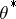via an equation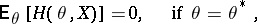whereis known, but the distribution ofis unknown and may or may not depend on(as indicated by the subscript on). In many situations,is the gradient of a functional to be minimized.

The general structure considered for stochastic algorithms is the following: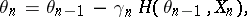where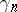is a non-negative non-increasing sequence, typicallyor a constant,is the "somehow stationary" observed sequence and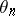is at eachan improved estimate of.

Note that when, and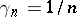,is simply the arithmetic mean of the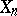.

The easiest situation is whenis a given sequence of independent identically-distributed random variables. However, this is not always the case: the classical Robbins–Monro algorithms correspond to the situation where a parameter(e.g., a dosage of a chemical product) needs to be tuned so that the effect measured byis at an average given level. In this caseandis the result of an experiment made with. Study of stochastic algorithms is generally restricted to those which have the Markovian structure introduced in [a1], whereis a random variable depending only on; more precisely, the distribution ofconditional to the whole pastis given by a transition kernel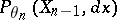. In this case, the expectation above has to be taken with respect to the stationary distribution of the Markov chainwhenis fixed.

## Decreasing gain.

In the case of decreasing gain, typical results which are proved are the almost-sure convergence ofto a solution of the equation(where), and, if, the asymptotic normality of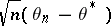and the law of the iterated logarithm ([a3], [a6], [a1], [a4]). The asymptotic covariance matrixofis the solution of the Lyapunov equationwhere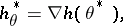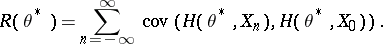The expectation is taken with respect to the distribution of the sequence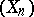(in the general Markovian situation, one has to consider here the stationary distribution of the chain of transition kernels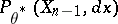). In particular, asymptotic normality occurs only if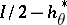has only negative eigenvalues (otherwise the Lyapunov equation above has no solution). Improving this covariance may be done by insertion of a matrix gain (smallest),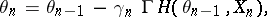and a simple computation shows that the optimal gain isThis matrix may be estimated during the algorithm, but convergence becomes quite difficult to prove. With this choice of the gain, the Cramér–Rao bound is attained. Another way is to use the Polyak–Ruppert averaging method [a7]: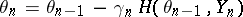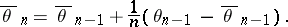with a "larger"(typically). One can prove the asymptotic optimality of this algorithm.

## Constant gain.

Constant-gain algorithms are used for tracking a slowly varying optimal parameter. The asymptotic behaviour of the algorithm may be studied when the speed of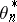andtend to zero [a1]; this so-called method of diffusion approximation consists in proving that, ifis fixed, then the trajectory of, suitably centred and rescaled in space and time, is well approximated by a diffusion. One shows that in the case of a smooth variation of, the gain has to be tuned approximately proportional to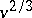, where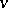is the speed of variation of. On the other hand, ifmoves along a random walk, the gain has to be chosen proportional to the average amplitude of. Other authors study the stationary limit distribution ofwhenhas a given distribution ([a2] and references therein). The direct on-line estimation of a good gainwithout a priori knowledge on the variation ofremains an important open problem.